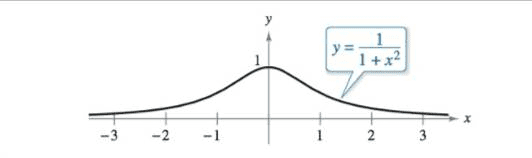### Create an Account

Home / Questions / Tangent Lines Find the point on the graph of (see Figure) where the tangent line has t...

# Tangent Lines Find the point on the graph of (see Figure) where the tangent line has the greatest slope, and the point where the tangent line has the least slope. Chapter 3, Problem 13PS, Tangent

Tangent Lines

Find the point on the graph of(see Figure) where the tangent line has the greatest slope, and the point where the tangent line has the least slope.Aug 04 2021 View more View LessSubscribe To Get Solution